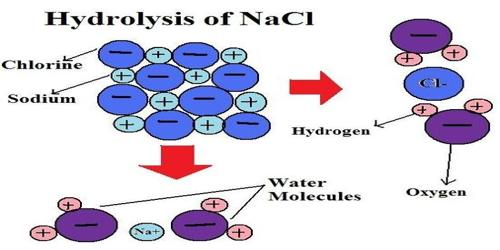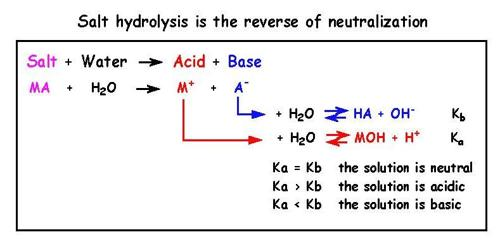# Salt Hydrolysis

Salt Hydrolysis

Salts are ionic compounds produced by the neutralization of acids with bases. Solutions of salts in water are expected to be neutral with a pH of 7.0, but in many cases, depending on the acid and base involved in the neutralization reaction, the solution is either acidic or basic. As will be shown below this happens because one or more of the ions which are present in such solutions react with water. Reactions of salts with water are known salt hydrolysis.

Salts prepared from strong acids and strong bases are neutral with a pH = 7.0. Salts from the neutralization of weak acids by strong bases give an alkaline solution of pH > 7.0, while salts formed from strong acids and weak bases are acidic in nature at pH < 7.0.

For understanding the phenomenon of salt hydrolysis use may be made of the Bronsted – Lowry concept of acids and bases which suggests that some ions act as acids or bases. For example when HCl gives ions in solution we write-

HCl (aq) → H+ (aq) + Cl (aq)

Here Cl is the conjugate base of the acid HCl. It is a strong acid Cl is a very weak base. In CH3COOH, however, C’H3COO is a relatively strong (conjugate) base as the acid is weak.In a solution of a salt of a strong acid and a strong base, e.g., NaCl, Na+ and Cl ions do not react with water as they are very weak acid and weak base respectively. It may be seen that it is the strong conjugate base of a weak acid and the strong conjugate acid of a weak base that hydrolyze, i.e., undergo reaction with water.

The hydrolysis of sodium ethanoate may now be explained. Ethanoate ion, C’H3COO react with water as follows:

C’H3COO (aq) + H2O (l) ↔ CH3COOH (aq) + OH (aq)

As OH is a stronger base and CH3COOH is a weak acid a solution of sodium ethanoate is alkaline.

Another familiar example is that of a solution of Na2CO3. CO32- is a strong conjugate base of the weak acid H2CO3. As a result of aqueous solution CO32- ion reacts with water as follows:

CO32- + H2O ↔ HCO3 + OH

OH being a strong base and HCO3 a weak acid the solution of Na2CO3 is alkaline.

If we consider a solution of NH4Cl we may note that NH4+ will react with water as follows:

NH4+ (aq) + H2O (l) ↔ NH4OH (aq) + H+ (aq)

Since H+ is a strong acid and NH4OH is a weak base the solution of NH4Cl is slightly acidic.

If the salt is formed from a weak acid and a weak base, for instance, ammonium ethanoate, then both NH4+ and CH3COO ions react with water as follows:

NH4 (aq) + H2O (l) ↔ NH4OH (aq) + H+ (aq)

CH3COO + H2O (l) ↔ CH3COOH (aq) + OH (aq)